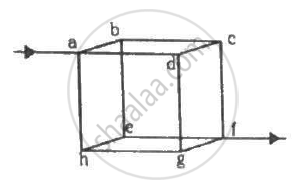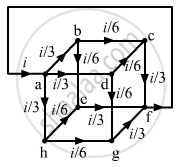Department of Pre-University Education, KarnatakaPUC Karnataka Science Class 12
Advertisement Remove all ads

# Twelve Wires, Each of Equal Resistance R, Are Joined to Form a Cube, as Shown in the Figure. Find the Equivalent Resistance Between the Diagonally-opposite Points A And F. - Physics

Sum

Twelve wires, each of equal resistance r, are joined to form a cube, as shown in the figure. Find the equivalent resistance between the diagonally-opposite points a and f.Advertisement Remove all ads

#### Solution

Let V be the potential difference between the points a and f. Let current i enter a and leave from f. The distribution of current in various branches is shown in figure.To calculate the potential difference between a and f, consider the path abcf and apply Kirchofff's Law:-

$\frac{i}{3}r + \frac{i}{6}r + \frac{i}{3}r = V$

$\Rightarrow \left( \frac{2ir}{3} + \frac{ir}{6} \right) = V$

$\Rightarrow \left( \frac{5ir}{6} \right) = V$

The effective resistance between a and f,

$R_{eff} = \frac{V}{i} = \frac{5}{6}r$

Is there an error in this question or solution?
Advertisement Remove all ads

#### APPEARS IN

HC Verma Class 11, Class 12 Concepts of Physics Vol. 2
Chapter 10 Electric Current in Conductors
Q 44 | Page 201
Advertisement Remove all ads

#### Video TutorialsVIEW ALL 

Advertisement Remove all ads
Share
Notifications

View all notifications

Forgot password?